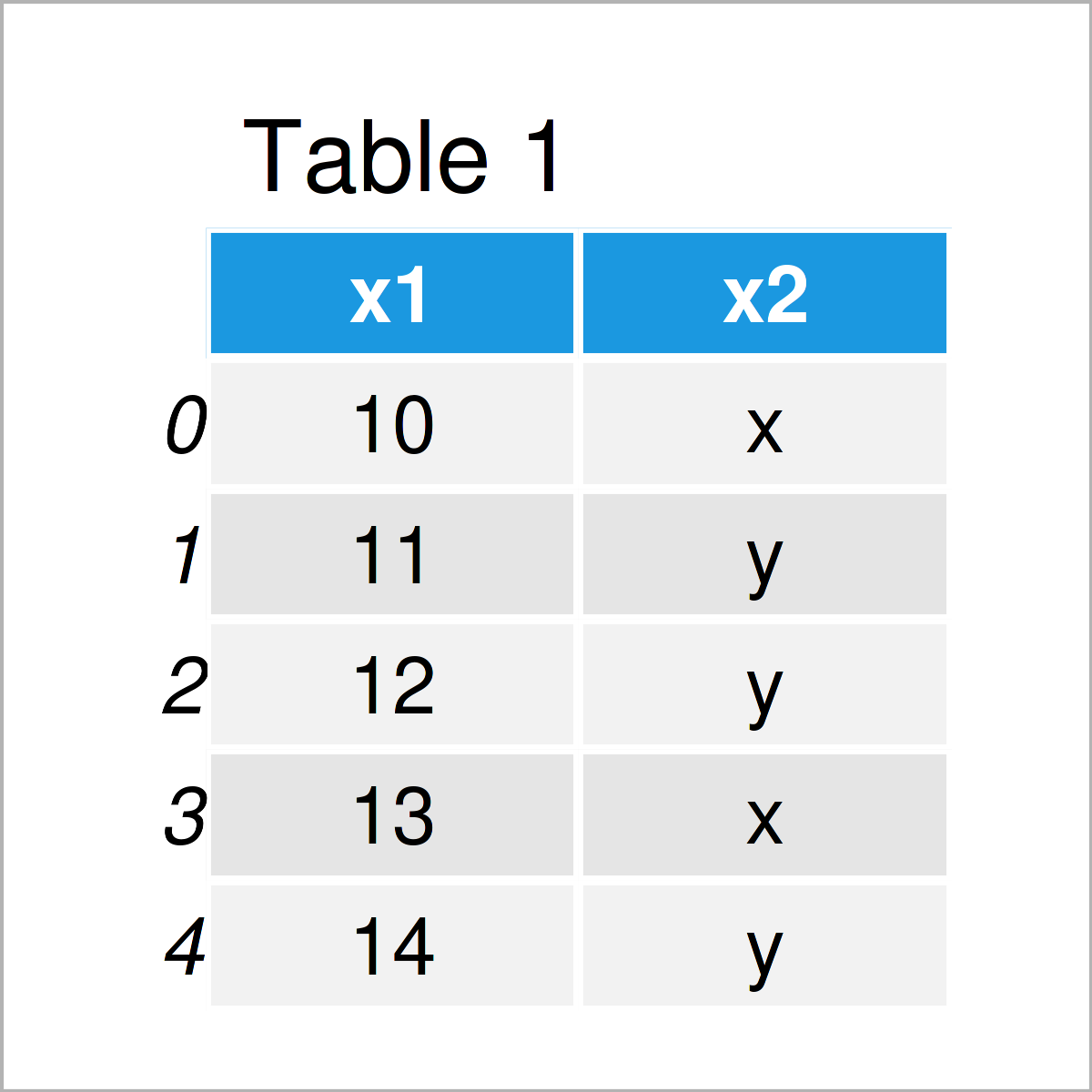# Create 1/0 Dummy Variable in pandas DataFrame in Python (2 Examples)

This tutorial demonstrates how to convert a pandas DataFrame column to a 1/0 dummy matrix in Python programming.

The article contains the following topics:

Here’s how to do it…

## Exemplifying Data & Libraries

To be able to use the functions of the pandas library, we first have to load pandas:

`import pandas as pd                           # Import pandas library in Python`

Furthermore, consider the following example data:

```data = pd.DataFrame({'x1':range(10, 15),      # Create example DataFrame
'x2':['x', 'y', 'y', 'x', 'y']})
print(data)                                   # Print example DataFrame```Table 1 shows that our example data has five observations and two columns.

## Example 1: Convert pandas DataFrame Column to Dummies Using get_dummies() Function

In Example 1, I’ll explain how to create a dummy matrix based on the values in a categorical variable.

For this task, we can apply the get_dummies function as shown below:

```data_dummies = pd.get_dummies(data['x2'])     # Convert column to dummies
print(data_dummies)                           # Print dummies```The output of the previous code is shown in Table 2: We have constructed a matrix containing a dummy column for each of the categories in our input variable.

## Example 2: Combine Dummies & pandas DataFrame

This example shows how to concatenate our example DataFrame with the dummy matrix that we have created in Example 1.

To accomplish this, we can apply the concat function:

```data_all = pd.concat([data, data_dummies],    # Combine original data & dummies
axis = 1)
print(data_all)                               # Print all data```By executing the previous Python syntax, we have created Table 3, i.e. a union of our example data and our dummy matrix.

## Video & Further Resources

Please accept YouTube cookies to play this video. By accepting you will be accessing content from YouTube, a service provided by an external third party.If you accept this notice, your choice will be saved and the page will refresh.

Furthermore, you could read the related articles that I have published on my website. I have released numerous other tutorials already: